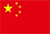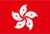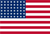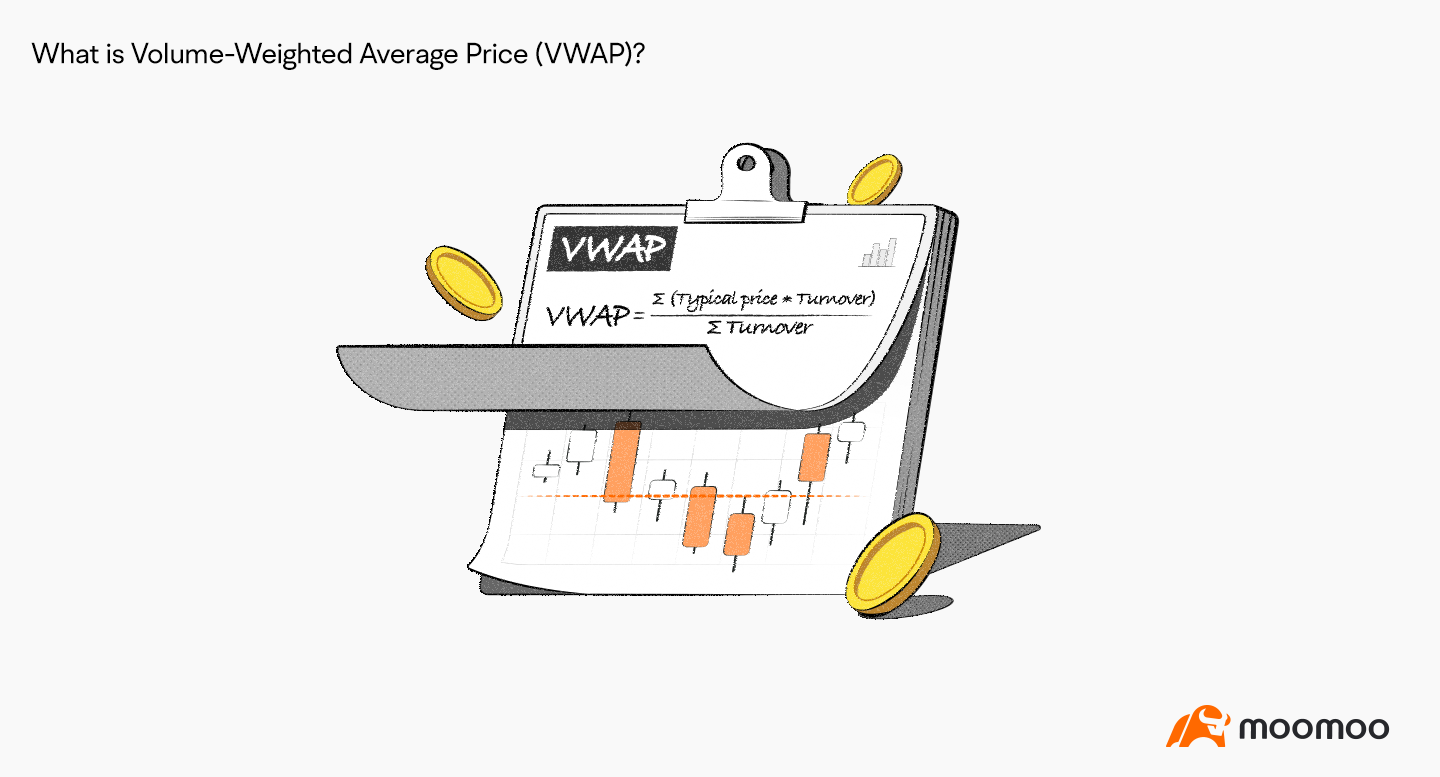Scanning QR Code• Online Inquiry

+
Congratulations
You've successfully got advanced quotes worth 5000 HKD
• Home
• Quotes
• News
• Community
• Learn
• Help Center
• Language
•••EN

# What Is A Volume-weighted Average Indicator?

Views 14342022.12.09# Key Takeaways

● Volume-Weighted Average Price(VWAP) measures the average asset price weighted by the total trading volume.

● VMAP is usually used on intraday charts.

● The current stock price is on the rise if it moves above the VMAP.

# Understanding VWAP

Volume-Weighted Average Price (VWAP) is a technical analysis tool to weight the average asset price by the total trading volume.

# How to calculate VWAP

The basic formula for VWAP is as follows:

VWAP = ∑ (Typical Price * Volume) / ∑ Volume

Here, Typical Price = (High Price + Low Price + Closing Price) / 3.

# How to use VMAP

VMAP is usually used on intraday charts to ascertain the basic intraday price moves.

VMAP is similar to SMA (Simple Moving Average).

When the stock price moves above the VMAP, it sends a bullish signal, where the current stock price is on the rise.

By contrast, when the stock price falls and moves below the VMAP, it suggests that the stock is in a downtrend.

# Summary

VAWP reflects the average asset price relative to the trading volume within a specific time period.

As a lagging indicator, however, VAWP is unable to predict future prices.